International
Tables for
Crystallography
Volume C
Mathematical, physical and chemical tables
Edited by E. Prince

International Tables for Crystallography (2006). Vol. C, ch. 9.8, pp. 913-915

## Section 9.8.2. Outline for a superspace-group determination

T. Janssen,a A. Janner,a A. Looijenga-Vosb and P. M. de Wolffc

aInstitute for Theoretical Physics, University of Nijmegen, Toernooiveld, NL-6525 ED Nijmegen, The Netherlands,bRoland Holstlaan 908, NL-2624 JK Delft, The Netherlands, and cMeermanstraat 126, 2614 AM, Delft, The Netherlands

### 9.8.2. Outline for a superspace-group determination

| top | pdf |

In the case of a modulated structure, the diffraction pattern consists of main reflections and satellites. The main reflections span a reciprocal lattice generated by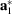,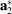,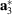. Considerations are here restricted for simplicity to the one-dimensional modulated case, i.e. to the n = 4 case. Extension to the more general n = 3 + d case is conceptually not difficult and does not modify the general procedure outlined here.

(1) The first step is the determination of the Laue group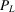of the diffraction pattern: it is the point group in three dimensions that transforms every diffraction peak into a peak of the same intensity.1

Asleaves invariant the subset of main reflections, this Laue group belongs to one of the 11 Laue symmetry classes. Accordingly, the Laue group determines a three-dimensional holohedral point group which determines a crystallographic system.

(2) The second step consists of choosing a basis according to the conventions of ITAfor the main reflections and choosing a modulation wavevector.

From the centring extinctions, one can deduce to which Bravais class the main reflections belong. This is one of the 14 three-dimensional Bravais classes. Notice that the cubic Bravais classes do not occur because a one-dimensional (incommensurate) modulation is incompatible with cubic symmetry. For this same reason, only the nine non-cubic Laue-symmetry classes occur in the one-dimensional incommensurate case.

The main reflections are indexed by hkl0 and the satellite reflections by hklm. The Fourier wavevector of a general reflection hklm is given by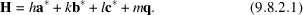Note that this step involves a choice because the system of satellite reflections is only defined modulo the main reflections. When a satellite is in the vicinity of a main reflection, it is reasonable to assign it to that reflection. But one has, especially when deciding whether or not situations are equivalent, to be aware of the fact that each satellite may be assigned to an arbitrary main reflection. It is even possible to assign a satellite to an extinct main reflection. One takes by preference the q vector along a symmetry axis or in a mirror plane. According to equation (9.8.2.1), the fourth basis vector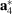is equal to the chosen q, the modulation wavevector.

(3) In the third step, one determines the space group of the average structure (from the main reflections).

The average structure is unique but possibly involves split atoms. The space group of the average structure is often the symmetry group of the undistorted phase. That helps to make a good choice for the basic structure and also gives an insight as to how the satellite reflections split from the main reflections at the phase transition.

(4) Step four is the identification of the (3 + 1)-dimensional Bravais lattice type. In superspace also, centring gives rise to centring extinctions, and that corresponds to making the choice of a conventional unit cell in (3 + 1) dimensions.

The previous three steps establish a*, b*, c*, the three-dimensional Bravais class and q = αa* + βb* + γc*, where the components α, β, and γ are given with respect to the three-dimensional conventional basis.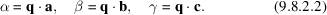The (3 + 1)-dimensional Bravais class is fixed by that three-dimensional Bravais class and the components α, β, γ of q.

Just as for three-dimensional lattices, a conventional cell can be chosen for (3 + 1)-dimensional lattices. To this end, the vector q must be considered. The vector q can be split into an invariant (or irrational) and a rational component according to: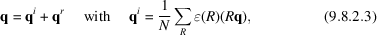where summation is over the elements R of the Laue group, N is the order of, and= ±1 follows from the property (valid in the one-dimensional modulated case):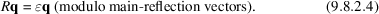If the splitting (9.8.2.3)results in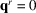, there is no problem. For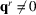we choose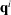as the new modulation wavevector. This may cause satellites to be assigned to extinct main reflections, or even to `main reflections' with non-integer indices. In this case, it is desirable to choose a new basissuch that all reflections can be written as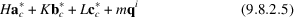with H, K, L, and m integer numbers. The basis obtained is the conventional basis for the M* module and corresponds to the choice of a conventional cell for the (3 + 1)-dimensional Bravais lattice.

Observed (possible) centring conditions for the reflections can be applied to determine the (3 + 1)-dimensional Bravais class.

Example 1. As an example, consider a C-centred orthorhombic lattice with modulation wavevector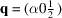, or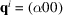and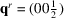. The general reflection condition is: hklm, h + k = even. A reflection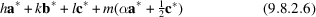can be described on a conventional basis with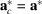,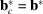,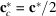as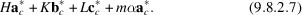Because H = h, K = k, L/2 = l + m/2, and h, k and l are integers with h + k even, one has the conditions H + K = even and L + m = even. The adopted conventional basis has centring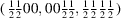. The conditions H + K = even and L + m = even become, after interchange of H and L, K + L = even and H + m = even, which according to Table 9.8.3.6gives mmmA(½0γ). Changing back to the original setting gives the Bravais class mmmC(α0½).

Example 2. As a second example, consider a C-centred orthorhombic lattice of main reflections. Suppose that an arbitrary reflection is given by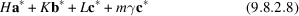with respect to the conventional basis a*, b*, c* of the C-centred lattice and that the general reflection condition is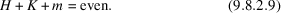This is in agreement with the C-centring condition H + K = even for the main reflections. Equation (9.8.2.9)implies a centring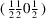of the (3 + 1)-dimensional lattice. Its conventional basis corresponding to (9.8.2.8)is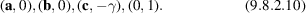Because of the centring translation [(a + b)/2, 1/2], a primitive basis ofis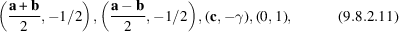which corresponds, according to (9.8.1.11), to a choice q = a*+ γc*. In terms of this modulation vector, an arbitrary reflection (9.8.2.8)can be described as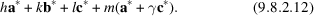Because H = h + m, K = k, and L = l, the reflection condition (9.8.2.9)now becomes h + k = even, again in agreement with the fact that the lattice of main reflections is C-centred.

(5) Step five: one lists all point groups (more precisely, the arithmetic crystal classes) compatible with the external Bravais class (i.e. the three-dimensional one), the components of the modulation vector q with respect to the conventional basis of this class, and the observed general extinctions. These groups are listed in Table 9.8.3.3.

The external part of the (3 + 1)-dimensional point group does not need to be the same as the point group of the average structure: it can never be larger, but is possibly a subgroup only.

(6) Step six consists of finding the superspace groups compatible with the previously derived results and with the special extinctions observed in the diffraction pattern.

For each arithmetic point group, the non-equivalent superspace groups are listed in Table 9.8.3.5.

Example 3. Continue with example 2of step 4. The external Bravais class of the modulated crystal is mmmC and q = (10γ). Consider the two cases of special reflection conditions.

 (1) The observed condition is: 00Lm, m = 2n. Then there is only one superspace group that obeys this condition: No. 21.4 with symbol C222(10γ)00s in Table 9.8.3.5. [See Subsection 9.8.3.3for explanation of symbols; note that C222 denotes the external part of the (3 + 1)-dimensional space group.] (2) There is no special reflection condition. Possible superspace groups are: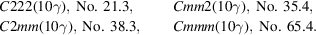In this second case, there are thus four possible superspace groups, three without and one with inversion symmetry. The difficulty stems from the fact that (in the absence of anomalous dispersion) the Laue group always has an inversion centre. Just as in three-dimensional crystallography, the ambiguity can only be solved by a complete determination of the structure, or by non-diffraction methods.

In the determination of modulated structures, one generally starts with the assumption that the external part of the (3 + 1)-dimensional point group is equal to the point group of the basic structure. This is, however, not necessarily true. The former may be a subgroup of the latter. Especially when the basic structure contains atoms at special positions, the point-group symmetry may be lowered by the modulation.

(7) Step seven concerns the restrictions imposed on/by the displacive or occupation modulation waves. For each occupied special Wyckoff position of the basic structure, one has to verify the validity of the restrictions imposed by the elements of the superspace group on the modulation as expressed in equation (9.8.1.24).

During the structure determination, one should check for each Wyckoff position which is occupied by an atom of the basic structure, whether the assumed displacive or occupation modulations obey the site symmetry of the Wyckoff position considered. An atom at a special position transforms into itself by the site symmetry of the position. For such a symmetry transformation,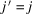, v = 0, and thus Δ(= 1) = τ, Δ(= −1) = 0 (cf. Subsection 9.8.3.3). In the modulated structure, the site symmetry is preserved only if, for each of its symmetry operations, the appropriate relation is obeyed by the modulations [cf. (9.8.1.24)].

For displacive modulations, the conditions are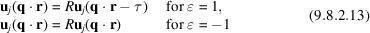and, for occupation modulation,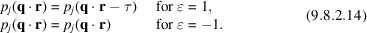Example 4. Assume, as for the case discussed above, that the basic structure has the space group Cmmm. Can the superspace group be Cmmm(10γ)? In this superspace group, τ = 0 for all symmetry operations with= 1. Displacive modulations at special positions must thus obey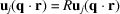for the superspace group to be correct. For an atom at special position mmm, this is not possible for all site symmetry operations unless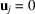. Suppose that the structure model contains a displacive modulation polarized along a for that atom. The allowed site symmetry is then lowered to 2mm, and as a consequence the superspace group is C2mm(10γ) rather than Cmmm(10γ).

### References

International Tables for Crystallography (2005). Vol. A, edited by Th. Hahn, fifth ed. Heidelberg: Springer.